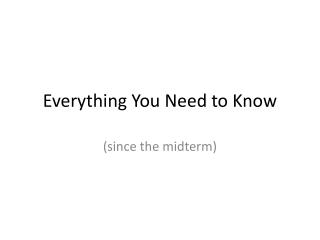DownloadDownload PresentationEverything You Need to Know

# Everything You Need to Know

Télécharger la présentation## Everything You Need to Know

- - - - - - - - - - - - - - - - - - - - - - - - - - - E N D - - - - - - - - - - - - - - - - - - - - - - - - - - -
##### Presentation Transcript

1. Everything You Need to Know (since the midterm)

2. Diagnosis • Abductive diagnosis: a minimal set of (positive and negative) assumptions that entails the observations • Consistency-based diagnosis: a minimal set of positive abnormality assumptions that is consistent with the observations. (Ab's not in the diagnosis are assumed to be false.)

3. Diagnosis • Abductive diagnoses: { Ab(a), Ab(b) } • Consistency-based diagnoses: { Ab(a) } and { Ab(b) }

4. SAT-Modulo Theories • Idea: propositions can be arithmetic constraints

5. SAT-Modulo Theories P1 Q P2 P3 Inconsistent! Learn (~P1 v ~P2 v ~P3)

6. Approximate Inference • One technique for approximate inference is to compute upper and lower bounds on a theory, where the bounds are in a restricted subset of logic that is tractable • Horn bounds: • There is a unique Horn LUB, equivalent to all the Horn clauses entailed by the theory • There can be many Horn GLBs, each is a weakest set of Horn clauses that entails the theory

7. Approximate Inference

8. Approximate Inference

9. Approximate Inference • Answering query F using bounds: • If F is Horn, then T|=F iff LUB|=F • Else: if LUB|=F, then T|=F • Else: if for all GLB L, L|=/=F, then T|=/=F • Else: we cannot tell if query is entailed by original theory by using the bounds

10. Multiple Agents • "Modal logic" extends FOL by including predicates whose arguments are formulas rather than terms • We can use it to represent the beliefs of different agents

11. Multiple Agents • The semantics of modal logic is based on "structures": a set of possible worlds, and a reachability relationship between worlds • An agent A believes P in world wiff P is true in all worlds w' reachable from w by the A relation In w1 A believes P? YES A believes ~Q? NO A believes B believes ~Q? YES A A A B P ~Q P Q w1 B w2

12. Bayesian Networks • A Bayesian network can be encoded in logic by introducing propositions that represent independent random (biased) coin flips • The probability of a model is the probability of the particular set of coin flips in the model • By weighting models by their probability, probabilistic inference becomes weighted model counting

13. Limits of FOL • FOL is the strongest logic with a complete proof theory • However, many commonplace mathematical notions cannot be expressed in FOL • For example, we cannot write a formula that says"P(x,y) is precisely the transitive closure of Q(x,y)"if Q is infinite • It can be useful in practice to use logics that are more expressive than FOL, even if they do not have complete proof theories • In practice, you give up a proof when you run out of time, even if the proof theory is complete.

14. Gödel • But things are even worse for logic than the limits of its expressivity • There are mathematical theorems that can be expressed in FOL, and that are demonstratively true, but for which no FOL proof exists. • Because FOL is complete, this means they are true but not entailed by the semantics of FOL either • That is Gödel's famous construction

15. Limits of Logic • Since FOL is the strongest complete logic, this means that there is no way around the limitation by finding a "stronger" logic • Another general limitation of logic discovered by Gödel is that any logic that is strong enough to be able to prove its own consistency must be inconsistent